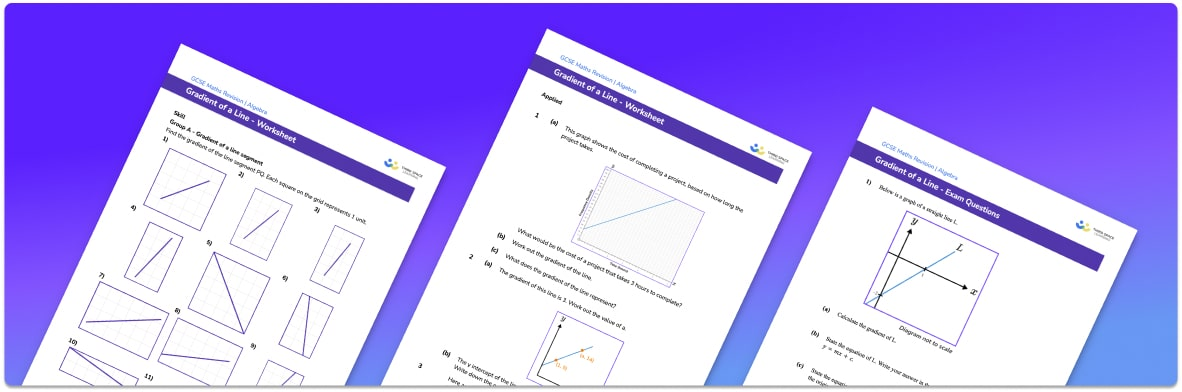# Straight Line Graphs Worksheet• Section 1 of the straight line graph worksheet contains 20+ skills-based straight line graph questions, in 3 groups to support differentiation
• Section 2 contains 3 applied straight line graph questions with a mix of worded problems and deeper problem solving questions
• Section 3 contains 3 foundation and higher level GCSE straight line graph exam style questions
• Answers and a mark scheme for all straight line graph questions are provided
• Questions follow variation theory with plenty of opportunities for students to work independently at their own level
• All questions created by fully qualified expert secondary maths teachers
• Suitable for GCSE maths revision for AQA, OCR and Edexcel exam boards

• This field is for validation purposes and should be left unchanged.

You can unsubscribe at any time (each email we send will contain an easy way to unsubscribe). To find out more about how we use your data, see our privacy policy.

### Straight line graph at a glance

Straight line graphs are graphs of linear functions and are often called linear graphs. Linear functions are functions involving x and y (with no powers).

The equation of a straight line is usually given in the form y=mx+c. When written in this way, m in the equation of the line is the gradient of the line and c is the y intercept. The gradient of a straight line tells us how steep the line is – the bigger the gradient, the steeper the line. A line with a positive gradient slopes upwards and a line with a negative gradient slopes downwards. The y intercept of a line is the point at which the line crosses the y axis.

When drawing straight line graphs, we use the equation y=mx+c to calculate the coordinates of the points of the line and create a table of values. We can plot the corresponding x values and y values onto a set of axes and join them together.

Horizontal and vertical lines have slightly adapted equations. Lines of the form y=a (where a is a constant) are straight horizontal lines with a gradient of 0. Lines of the form x=a (where a is a constant) are straight vertical lines and have a gradient of ∞. Parallel lines have the same gradient and the gradients of perpendicular lines are the negative reciprocal of each other.

We can use the line, or information about the line, to find the equation of the straight line, the midpoint of the line and the length of the line.

Looking forward, students can then progress to additional straight line graphs worksheets and on to more algebra worksheets for example a simplifying expressions worksheet or simultaneous equations worksheet.For more teaching and learning support on Algebra our GCSE maths lessons provide step by step support for all GCSE maths concepts.

## Do you have KS4 students who need more focused attention to succeed at GCSE?There will be students in your class who require individual attention to help them succeed in their maths GCSEs. In a class of 30, it’s not always easy to provide.

Help your students feel confident with exam-style questions and the strategies they’ll need to answer them correctly with our dedicated GCSE maths revision programme.

Lessons are selected to provide support where each student needs it most, and specially-trained GCSE maths tutors adapt the pitch and pace of each lesson. This ensures a personalised revision programme that raises grades and boosts confidence.

Find out more